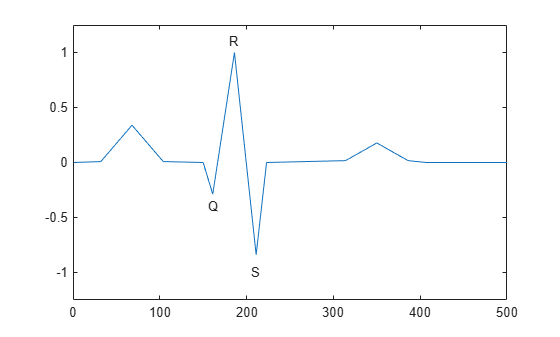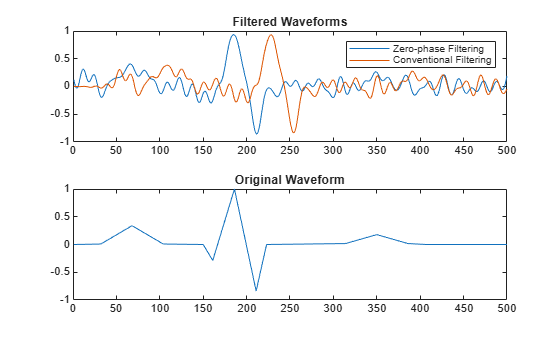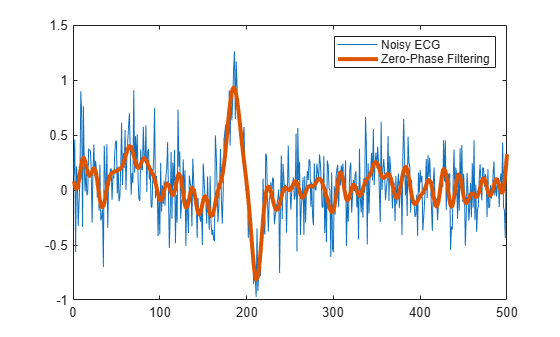Documentation

# filtfilt

Zero-phase digital filtering

## Syntax

``y = filtfilt(b,a,x)``
``y = filtfilt(sos,g,x)``
``y = filtfilt(d,x)``

## Description

example

````y = filtfilt(b,a,x)` performs zero-phase digital filtering by processing the input data, `x`, in both the forward and reverse directions. After filtering the data in the forward direction, `filtfilt` reverses the filtered sequence and runs it back through the filter. The result has the following characteristics: Zero phase distortion.A filter transfer function equal to the squared magnitude of the original filter transfer function.A filter order that is double the order of the filter specified by `b` and `a`. `filtfilt` minimizes start-up and ending transients by matching initial conditions. Do not use `filtfilt` with differentiator and Hilbert FIR filters, because the operation of these filters depends heavily on their phase response.```
````y = filtfilt(sos,g,x)` zero-phase filters the input data, `x`, using the second-order section (biquad) filter represented by the matrix `sos` and the scale values `g`.```
````y = filtfilt(d,x)` zero-phase filters the input data, `x`, using a digital filter, `d`. Use `designfilt` to generate `d` based on frequency-response specifications.```

## Examples

collapse all

Zero-phase filtering helps preserve features in a filtered time waveform exactly where they occur in the unfiltered signal.

Use `filtfilt` to zero-phase filter a synthetic electrocardiogram (ECG) waveform. The function that generates the waveform is at the end of the example. The QRS complex is an important feature in the ECG. Here it begins around time point 160.

```wform = ecg(500); plot(wform) axis([0 500 -1.25 1.25]) text(155,-0.4,'Q') text(180,1.1,'R') text(205,-1,'S')```Corrupt the ECG with additive noise. Reset the random number generator for reproducible results. Construct a lowpass FIR equiripple filter and filter the noisy waveform using both zero-phase and conventional filtering.

```rng default x = wform' + 0.25*randn(500,1); d = designfilt('lowpassfir', ... 'PassbandFrequency',0.15,'StopbandFrequency',0.2, ... 'PassbandRipple',1,'StopbandAttenuation',60, ... 'DesignMethod','equiripple'); y = filtfilt(d,x); y1 = filter(d,x); subplot(2,1,1) plot([y y1]) title('Filtered Waveforms') legend('Zero-phase Filtering','Conventional Filtering') subplot(2,1,2) plot(wform) title('Original Waveform')```Zero-phase filtering reduces noise in the signal and preserves the QRS complex at the same time it occurs in the original. Conventional filtering reduces noise in the signal, but delays the QRS complex.

Repeat the above using a Butterworth second-order section filter.

```d1 = designfilt('lowpassiir','FilterOrder',12, ... 'HalfPowerFrequency',0.15,'DesignMethod','butter'); y = filtfilt(d1,x); subplot(1,1,1) plot(x) hold on plot(y,'LineWidth',3) legend('Noisy ECG','Zero-Phase Filtering')```This is the function that generates the ECG waveform.

```function x = ecg(L) %ECG Electrocardiogram (ECG) signal generator. % ECG(L) generates a piecewise linear ECG signal of length L. % % EXAMPLE: % x = ecg(500).'; % y = sgolayfilt(x,0,3); % Typical values are: d=0 and F=3,5,9, etc. % y5 = sgolayfilt(x,0,5); % y15 = sgolayfilt(x,0,15); % plot(1:length(x),[x y y5 y15]); % Copyright 1988-2002 The MathWorks, Inc. a0 = [0,1,40,1,0,-34,118,-99,0,2,21,2,0,0,0]; % Template d0 = [0,27,59,91,131,141,163,185,195,275,307,339,357,390,440]; a = a0 / max(a0); d = round(d0 * L / d0(15)); % Scale them to fit in length L d(15)=L; for i=1:14, m = d(i) : d(i+1) - 1; slope = (a(i+1) - a(i)) / (d(i+1) - d(i)); x(m+1) = a(i) + slope * (m - d(i)); end end```

## Input Arguments

collapse all

Transfer function coefficients, specified as vectors. If you use an all-pole filter, enter `1` for `b`. If you use an all-zero (FIR) filter, enter `1` for `a`.

Example: `b = [1 3 3 1]/6` and ```a = [3 0 1 0]/3``` specify a third-order Butterworth filter with a normalized 3-dB frequency of 0.5π rad/sample.

Data Types: `double`

Input signal, specified as a real-valued or complex-valued vector, matrix, or N-D array. `filtfilt` operates along the first array dimension of `x` with size greater than 1.

Example: `cos(pi/4*(0:159))+randn(1,160)` is a single-channel row-vector signal.

Example: `cos(pi./[4;2]*(0:159))'+randn(160,2)` is a two-channel signal.

Data Types: `double`
Complex Number Support: Yes

Second-order section coefficients, specified as a matrix. `sos` is a K-by-6 matrix, where the number of sections, K, must be greater than or equal to 2. If the number of sections is less than 2, then `filtfilt` treats the input as a numerator vector. Each row of `sos` corresponds to the coefficients of a second-order (biquad) filter. The ith row of `sos` corresponds to ```[bi(1) bi(2) bi(3) ai(1) ai(2) ai(3)]```.

Example: `s = [2 4 2 6 0 2;3 3 0 6 0 0]` specifies a third-order Butterworth filter with a normalized 3-dB frequency of 0.5π rad/sample.

Data Types: `double`

Scale factors, specified as a vector.

Data Types: `double`

Digital filter, specified as a `digitalFilter` object. Use `designfilt` to generate a digital filter based on frequency-response specifications.

Example: ```d = designfilt('lowpassiir','FilterOrder',3,'HalfPowerFrequency',0.5)``` specifies a third-order Butterworth filter with a normalized 3-dB frequency of 0.5π rad/sample.

Data Types: `double`

## Output Arguments

collapse all

Filtered signal, returned as a vector, matrix, or N-D array.

 Oppenheim, Alan V., Ronald W. Schafer, and John R. Buck. Discrete-Time Signal Processing. 2nd Ed. Upper Saddle River, NJ: Prentice Hall, 1999.

 Mitra, Sanjit K. Digital Signal Processing. 2nd Ed. New York: McGraw-Hill, 2001.

 Gustafsson, F. “Determining the initial states in forward-backward filtering.” IEEE® Transactions on Signal Processing. Vol. 44, April 1996, pp. 988–992.